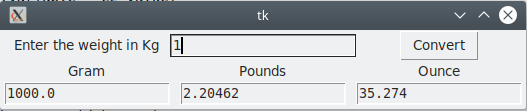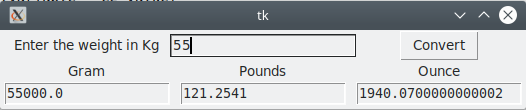# Python: Weight Conversion GUI using Tkinter

Prerequisites: Python GUI – tkinter

Python offers multiple options for developing a GUI (Graphical User Interface). Out of all the GUI methods, `tkinter` is the most commonly used method. It is a standard Python interface to the Tk GUI toolkit shipped with Python. Python with tkinter outputs the fastest and easiest way to create GUI applications. Creating a GUI using tkinter is an easy task.

Steps to Create a Tkinter:

• Importing the module – `tkinter`
• Create the main window (container)
• Add any number of widgets to the main window
• Apply the event Trigger on the widgets.

Let’s create a GUI based weight converter that accepts a kilogram input value and converts that value to grams, pounds, and ounces when the user clicks the Convert button.

Below is the implementation.

 `# Python program to  create a simple GUI   ` `# weight converter using Tkinter  ` ` `  ` `  `from` `tkinter ``import` `*` ` `  ` `  `# Create a GUI window ` `window ``=` `Tk() ` `  `  `# Function to convert weight ` `# given in kg to grams, pounds ` `# and ounces ` `def` `from_kg(): ` `     `  `    ``# convert kg to gram ` `    ``gram ``=` `float``(e2_value.get())``*``1000` `     `  `    ``# convert kg to pound ` `    ``pound ``=` `float``(e2_value.get())``*``2.20462`  `     `  `    ``# convert kg to ounce ` `    ``ounce ``=` `float``(e2_value.get())``*``35.274`  `     `  `    ``# Enters the converted weight to  ` `    ``# the text widget ` `    ``t1.delete(``"1.0"``, END) ` `    ``t1.insert(END,gram) ` `     `  `    ``t2.delete(``"1.0"``, END) ` `    ``t2.insert(END,pound) ` `     `  `    ``t3.delete(``"1.0"``, END) ` `    ``t3.insert(END,ounce) ` ` `  `# Create the Label widgets ` `e1 ``=` `Label(window, text ``=` `"Enter the weight in Kg"``) ` `e2_value ``=` `StringVar() ` `e2 ``=` `Entry(window, textvariable ``=` `e2_value) ` `e3 ``=` `Label(window, text ``=` `'Gram'``) ` `e4 ``=` `Label(window, text ``=` `'Pounds'``) ` `e5 ``=` `Label(window, text ``=` `'Ounce'``) ` ` `  `# Create the Text Widgets ` `t1 ``=` `Text(window, height ``=` `1``, width ``=` `20``) ` `t2 ``=` `Text(window, height ``=` `1``, width ``=` `20``) ` `t3 ``=` `Text(window, height ``=` `1``, width ``=` `20``) ` ` `  `# Create the Button Widget ` `b1 ``=` `Button(window, text ``=` `"Convert"``, command ``=` `from_kg) ` ` `  `# grid method is used for placing  ` `# the widgets at respective positions  ` `# in table like structure ` `e1.grid(row ``=` `0``, column ``=` `0``) ` `e2.grid(row ``=` `0``, column ``=` `1``) ` `e3.grid(row ``=` `1``, column ``=` `0``) ` `e4.grid(row ``=` `1``, column ``=` `1``) ` `e5.grid(row ``=` `1``, column ``=` `2``) ` `t1.grid(row ``=` `2``, column ``=` `0``) ` `t2.grid(row ``=` `2``, column ``=` `1``) ` `t3.grid(row ``=` `2``, column ``=` `2``) ` `b1.grid(row ``=` `0``, column ``=` `2``) ` ` `  `# Start the GUI  ` `window.mainloop() `

Output:My Personal Notes arrow_drop_upCheck out this Author's contributed articles.

If you like GeeksforGeeks and would like to contribute, you can also write an article using contribute.geeksforgeeks.org or mail your article to contribute@geeksforgeeks.org. See your article appearing on the GeeksforGeeks main page and help other Geeks.

Please Improve this article if you find anything incorrect by clicking on the "Improve Article" button below.

Article Tags :

1

Please write to us at contribute@geeksforgeeks.org to report any issue with the above content.